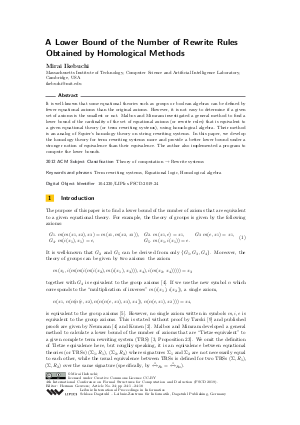Document# A Lower Bound of the Number of Rewrite Rules Obtained by Homological Methods

### Author Mirai Ikebuchi## File

LIPIcs.FSCD.2019.24.pdf
• Filesize: 0.6 MB
• 18 pages

## Cite As

Mirai Ikebuchi. A Lower Bound of the Number of Rewrite Rules Obtained by Homological Methods. In 4th International Conference on Formal Structures for Computation and Deduction (FSCD 2019). Leibniz International Proceedings in Informatics (LIPIcs), Volume 131, pp. 24:1-24:18, Schloss Dagstuhl - Leibniz-Zentrum für Informatik (2019)
https://doi.org/10.4230/LIPIcs.FSCD.2019.24

## Abstract

It is well-known that some equational theories such as groups or boolean algebras can be defined by fewer equational axioms than the original axioms. However, it is not easy to determine if a given set of axioms is the smallest or not. Malbos and Mimram investigated a general method to find a lower bound of the cardinality of the set of equational axioms (or rewrite rules) that is equivalent to a given equational theory (or term rewriting systems), using homological algebra. Their method is an analog of Squier’s homology theory on string rewriting systems. In this paper, we develop the homology theory for term rewriting systems more and provide a better lower bound under a stronger notion of equivalence than their equivalence. The author also implemented a program to compute the lower bounds.

## Subject Classification

##### ACM Subject Classification
• Theory of computation → Rewrite systems
##### Keywords
• Term rewriting systems
• Equational logic
• Homological algebra

## Metrics

• Access Statistics
• Total Accesses (updated on a weekly basis)
0

## References

1. D. Epstein. Finite presentations of groups and 3-manifolds. The Quarterly Journal of Mathematics, 12(1):205-212, 1961.2. K. Kunen. Single axioms for groups. Journal of Automated Reasoning, 9(3):291-308, December 1992. URL: http://dx.doi.org/10.1007/BF00245293.
3. P. Malbos and S. Mimram. Homological computations for term rewriting systems. In 1st International Conference on Formal Structures for Computation and Deduction (FSCD 2016), volume 52 of Leibniz International Proceedings in Informatics (LIPIcs), pages 27:1-27:17, Dagstuhl, Germany, 2016. Schloss Dagstuhl-Leibniz-Zentrum fuer Informatik.4. B. H. Neumann. Another single law for groups. Bulletin of the Australian Mathematical Society, 23(1):81–102, 1981. URL: http://dx.doi.org/10.1017/S0004972700006912.
5. B. H. Neumann. Yet another single law for groups. Illinois J. Math., 30(2):295-300, June 1986.6. J. J. Rotman. An Introduction to Homological Algebra. Springer-Verlag New York, 2009.7. J. J. Rotman. Advanced Modern Algebra, volume 114. American Mathematical Soc., 2010.8. C. C. Squier. Word problems and a homological finiteness condition for monoids. Journal of Pure and Applied Algebra, 49(1-2):201-217, 1987.9. A. Tarski. Equational Logic and Equational Theories of Algebras. In Contributions to Mathematical Logic, volume 50 of Studies in Logic and the Foundations of Mathematics, pages 275-288. Elsevier, 1968.X

Feedback for Dagstuhl Publishing